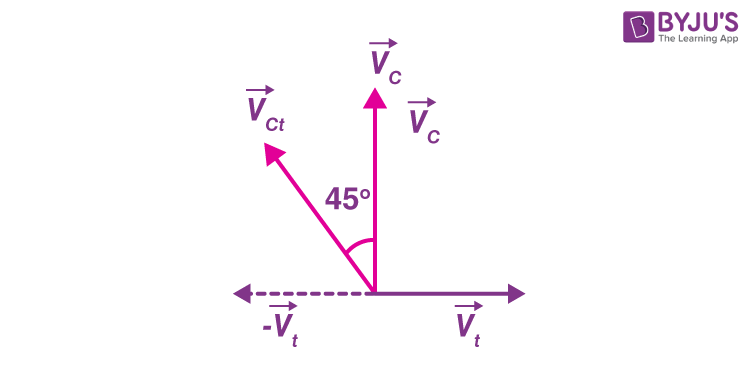# A train is moving due east and a car is moving due north with equal speeds. A passenger in the train finds that the car is moving towards

a) North – East

b) North – West

c) South – West

d) South – East

Solution:Let

$$\begin{array}{l}\vec{v_{c}}\end{array}$$
be the velocity of the car and
$$\begin{array}{l}\vec{v_{t}}\end{array}$$
be the velocity of the train.

We know that

$$\begin{array}{l}\vec{v_{ct}}=\vec{v_{c}}-\vec{v_{t}}\end{array}$$

Hence, from the figure, velocity of car w.r.t train (vct) is towards West – North.(0)(0)